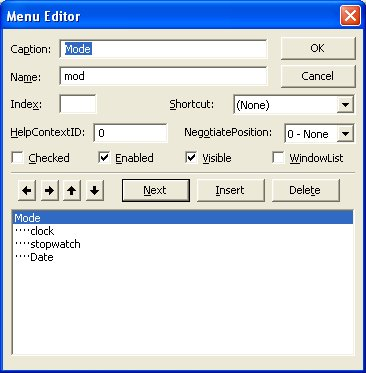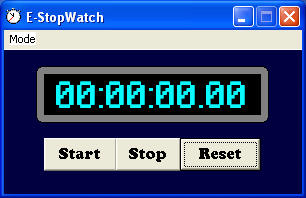# Digital Stopwatch

We can design a stopwatch that resembles a typical digital stopwatch. When the user clicks mode, he or she  can select clock, date or stopwatch. When he or she select clock, the current time is displayed and when date is selected, the current date will be displayed. Lastly, when the user select the stopwatch, all the digits will be set to 0. In this program, you need to insert one label, three command buttons and two timers. Timer1 is used for the stopwatch so we set the interval to 1(1000th of a second). Timer2 is used to display the clock so we set the interval to 1000(equivalent to 1 second). We also used  six string variables to display the digits of the stopwatch so that we can put in the colons ":" and the decimal point. We have also created a subroutine known as countime. To add the menu items to the interface, you need to add them from the menu editor, as shown in the Figure below:## The Runtime Interface### The code

Dim a As String
Dim b As String
Dim c As String
Dim x As String
Dim y As String
Dim z As String
Dim h As String
Dim m As String
Dim s As String
Dim u As String
Dim v As String
Public interval As Double

Private Sub clock_Click()
Timer1.Enabled = False
Timer2.Enabled = True
End Sub

Private Sub Command1_Click()
Timer1.Enabled = True
Timer1.interval = 1
End Sub

Private Sub Command2_Click()
Timer1.Enabled = False
End Sub

Private Sub Command3_Click()
Timer1.Enabled = False
a = "0"
b = "0"
c = "0"
x = "0"
y = "0"
z = "0"
u = "0"
v = "0"

h = a + b
m = c + x
s = y + z
'To set the display as "00:00:00.00"
Label1.Caption = h + ":" + m + ":" + s + "." + u + v
End Sub

Sub counttime()
If Val(v) < 9 Then
v = v + 1

Label1.Caption = a + b + ":" + c + x + ":" + y + z + "." + u + v
ElseIf Val(u) < 9 Then
v = 0
u = u + 1

Label1.Caption = a + b + ":" + c + x + ":" + y + z + "." + u + v
ElseIf Val(z) < 9 Then
v = 0
u = 0

z = z + 1

Label1.Caption = a + b + ":" + c + x + ":" + y + z + "." + u + v
ElseIf Val(y) < 5 Then
v = 0
u = 0
z = 0
y = y + 1
Label1.Caption = a + b + ":" + c + x + ":" + y + z + "." + u + v
ElseIf Val(x) < 9 Then
v = 0
u = 0
z = 0
y = 0
x = x + 1
Label1.Caption = a + b + ":" + c + x + ":" + y + z + "." + u + v
ElseIf Val(c) < 5 Then
v = 0
u = 0
z = 0
y = 0
x = 0
c = c + 1
Label1.Caption = a + b + ":" + c + x + ":" + y + z + "." + u + v

End If

End Sub

Private Sub Timer1_Timer()
counttime

End Sub

ElseIf Val(b) < 9 Then
v = 0
u = 0
z = 0
y = 0
x = 0
c = 0
b = b + 1
Label1.Caption = a + b + ":" + c + x + ":" + y + z + "." + u + v
ElseIf Val(b) < 9 Then
v = 0
u = 0
z = 0
y = 0
x = 0
c = 0
b = b + 1
Label1.Caption = a + b + ":" + c + x + ":" + y + z + "." + u + v
ElseIf Val(a) < 9 Then
v = 0
u = 0
z = 0
y = 0
x = 0
c = 0
b = 0
a = a + 1
Label1.Caption = a + b + ":" + c + x + ":" + y + z + "." + u + v

Private Sub date_Click()

Label1.Caption = Date
Timer2.Enabled = False

End Sub

a = "0"
b = "0"
c = "0"
x = "0"
y = "0"
z = "0"
u = 0
v = 0
h = a + b
m = c + x
s = y + z
'To set the display as "00:00:00.00"
Label1.Caption = h + ":" + m + ":" + s + "." + u + v

End Sub

Private Sub stopwc_Click()
Timer2.Enabled = False
a = "0"
b = "0"
c = "0"
x = "0"
y = "0"
z = "0"
u = "0"
v = "0"

h = a + b
m = c + x
s = y + z
Label1.Caption = h + ":" + m + ":" + s + "." + u + v

End Sub

Private Sub Timer2_Timer()
Label1.Caption = Time
End Sub